Examples

Chapter 13 Class 10 Statistics
Serial order wise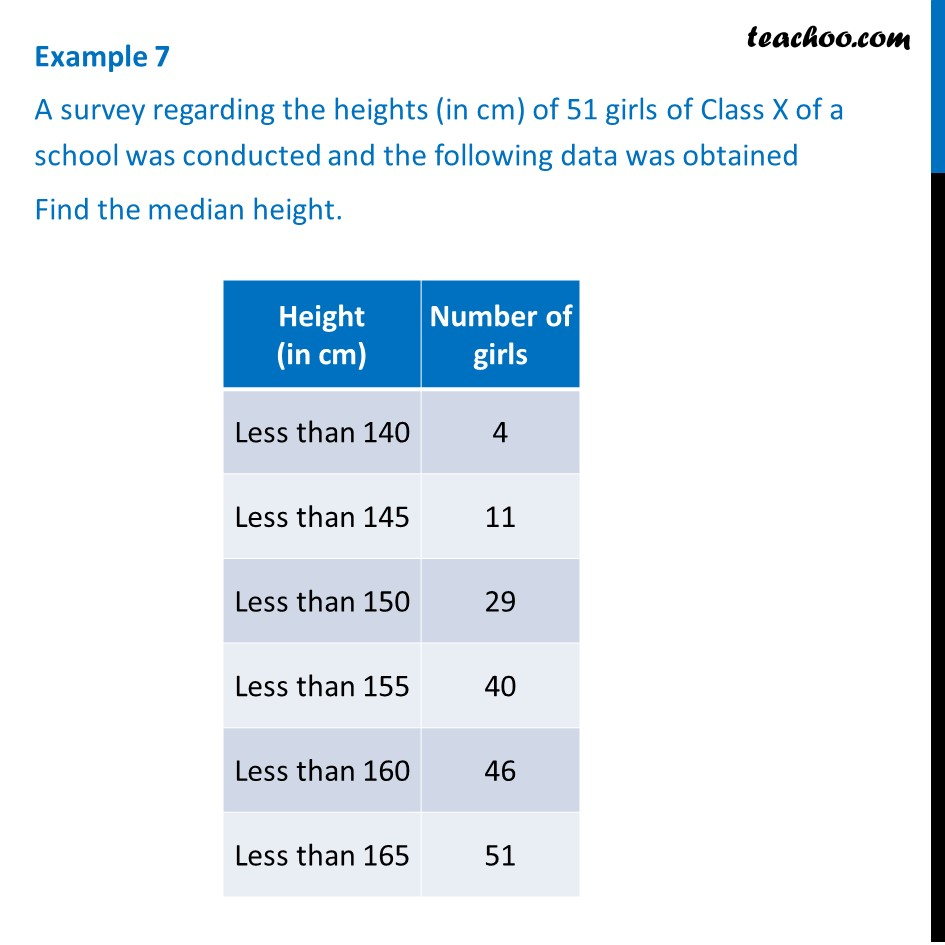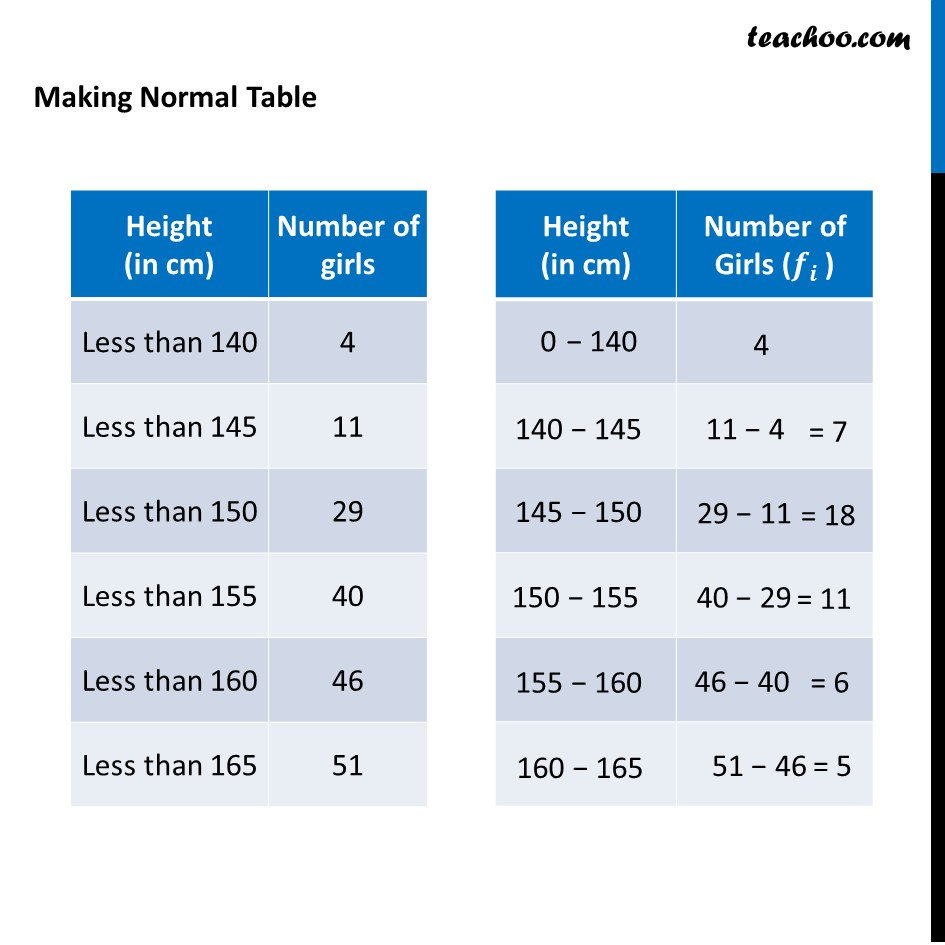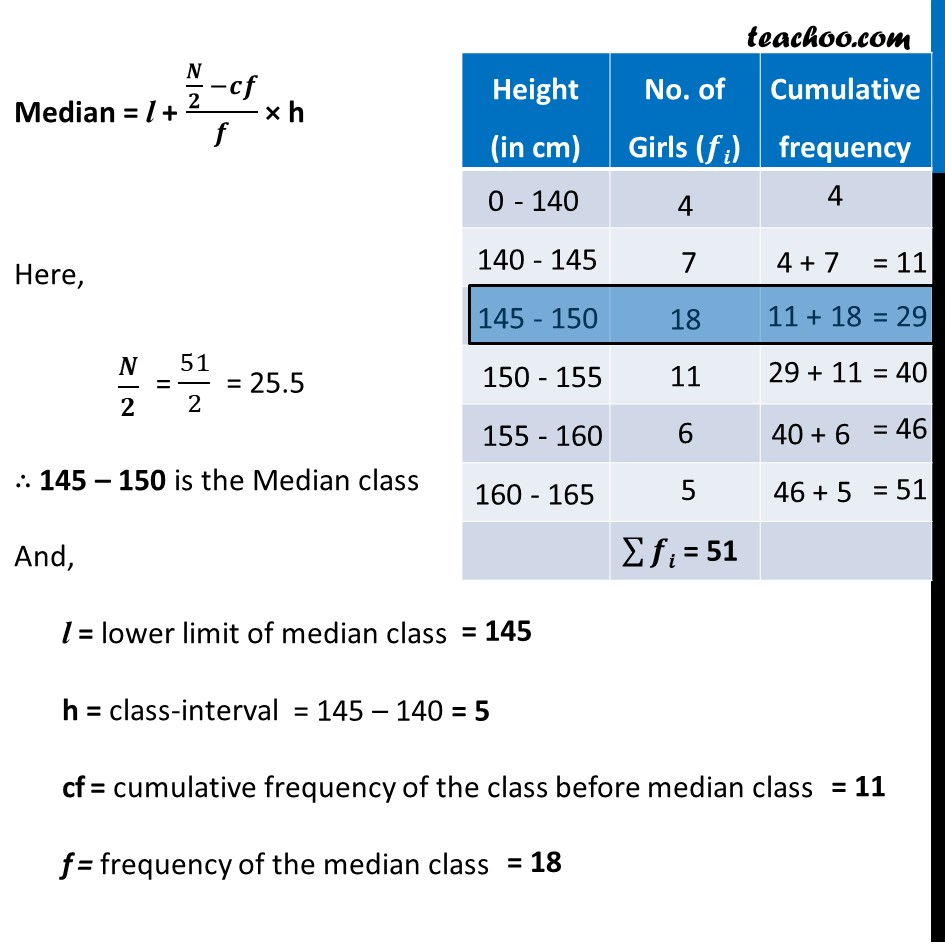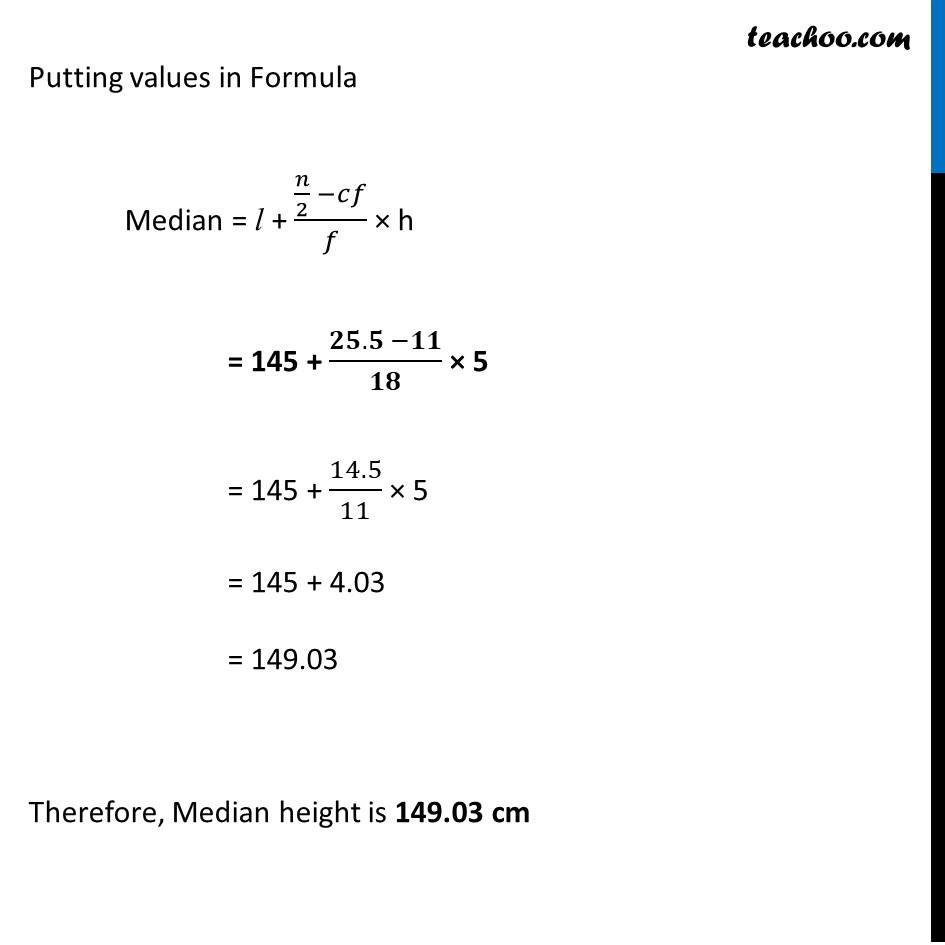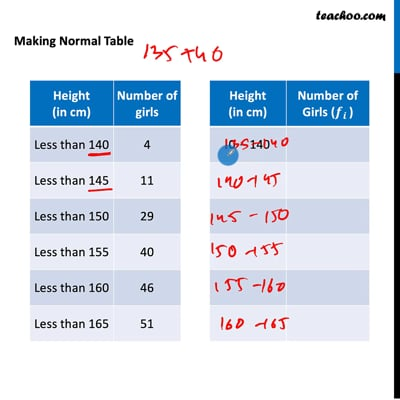This video is only available for Teachoo black users

Learn in your speed, with individual attention - Teachoo Maths 1-on-1 Class

### Transcript

Example 7 A survey regarding the heights (in cm) of 51 girls of Class X of a school was conducted and the following data was obtained Find the median height. Making Normal Table Median = l + (𝑵/𝟐 −𝒄𝒇)/𝒇 × h Here, 𝑵/𝟐 ∴ 145 – 150 is the Median class And, l = lower limit of median class h = class-interval cf = cumulative frequency of the class before median class f = frequency of the median class Putting values in Formula Median = l + (𝑛/2 −𝑐𝑓)/𝑓 × h = 145 + (𝟐𝟓.𝟓 −𝟏𝟏)/𝟏𝟖 × 5 = 145 + 14.5/11 × 5 = 145 + 4.03 = 149.03 Therefore, Median height is 149.03 cm